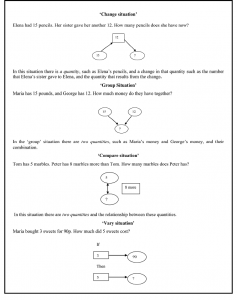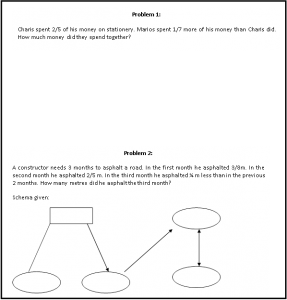# Scenario: Solving problems using Schema-Based Instruction

## Context – national, curricular, professional, other

Schema-Based Instruction aims to develop students’ understanding of the basic relations found in arithmetic word problems (Marshall, 1995). Students are taught to map features of word problems onto problem schemata. Figure 1 illustrates the four schemas that are included in mathematics textbooks in Cyprus used to describe the semantic relations found in story problems, namely, ‘change’, ‘group’, ‘compare’, and ‘vary’Figure 1: Schema- problems

## Scenario

Elsa, a final year university student in a teacher preparation programme, was teching problem solving using Schema-Based Instruction with a Year 5 (pupils age 10-11) class. The lesson took place in a school-based placement towards the end of her four-year teacher preparation.

Figure 2 illustrates two problems from an activity that Elsa developed for her lesson on Schema- Based instruction. The students were asked to complete a given diagram with the information necessary to solve a problem.Figure 2: Elsa’a suggested schema problems

# Knowledge Quartet Coding Commentary

## Scenario: Elsa teaching problem solving using schema-based instruction

In problem 1 (figure 2)  the first diagram provided for the story is correct. It represents a comparison situation needed to find the amount of money that Marios spent. The second diagram represents a group problem and is needed to find how much money Marios and Charis spent together. However, Elsa’s decision not to say how much money Charis and Marios had to start with proved problematic. It is possible that Elsa did this intentionally because she wanted the students to give an answer in the form of fractions. Even if this was the case the story needs to state that Marios and Charis had the same amount of money at the beginning.

In the second problem the first diagram given represents a change situation. Even though the situation in the problem could be seen as a change in the number of metres of road that were asphalted, the use of a group situation (the amount asphalted in the first month and the amount asphalted in the second month) would be more sensible. Elsa’s choice here could cause difficulties for pupils who are not yet confident in distinguishing a change from a group situation. (This seemed to be the case with some students in Elsa’s classroom). Elsa’s failure to see the potential problem stemmed from limited understanding of the schemas and also, due to her inability to anticipate students’ difficulties with the topic. A further problem is the choice of numbers in a supposedly real life situation. The choice of numbers was not realistic. In real life the constructor would asphalt more than 3/8 metres in a month. I asked Elsa why she had decided to use fractions in all her problems. She said that this was done because all the problems in the textbooks included fractions.  She added that she had tried to choose fractions that her students could add or subtract easily. However, it seems that Elsa did not take into consideration the fact that in some cases her choice of fractions was not an appropriate representation for her proposed problems. Therefore, it seems that she chose the numbers in the problems without giving them sufficient thought.

## References

Marshall, S.D. (1995) Schemas in problem solving, (Cambridge, Cambridge University Press).